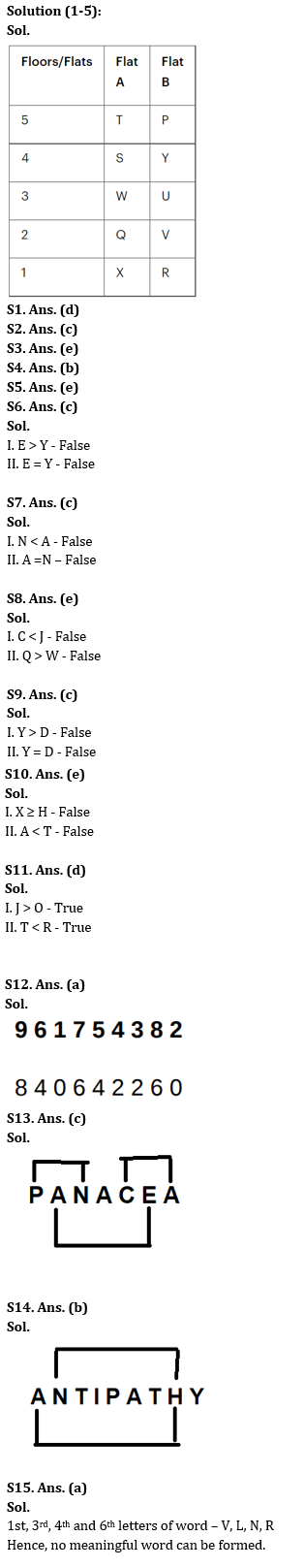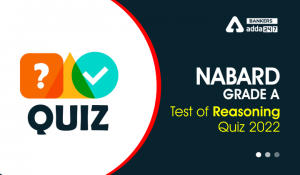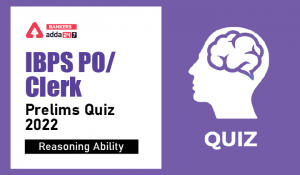Latest Banking jobs   »

# Reasoning Ability Quiz For IDBI AM/Executive 2022- 5th July

Directions (1-5): Answer the following questions based on the information given below.
There are two types of flats i.e., flat A and flat B on each floor of a five- floor building. The bottommost floor is numbered as 1, just above it is numbered as 2 and so on till the topmost floor is numbered as 5. Flat A is in west of Flat B. Ten persons (P, Q, R, S, T, U, V, W, X, and Y) live in these flats such that one person lives in each flat but not necessarily in the same order.
Note: If a person lives in a flat adjacent to another person, then both persons live on the same floor. If a person lives two/three floors above/below another person, then both persons live in the same type of flat unless stated otherwise.
Q doesn’t live in Flat B. U lives immediately above V. P lives two floors above W but not in the same type of flat. Y lives adjacent to S but not in the same type of flat as W. Both U and V live on a prime numbered floor. W lives two floors above X. Neither U nor V lives adjacent to X. Neither Q nor R lives above S. V doesn’t live adjacent to R.

Reasoning Quiz For IDBI AM/Executive 2022 5th July CHECK IN HINDI

Q1. T lives on which of the following floor?
(a) 4th floor
(b) 3rd floor
(c) 1st floor
(d) Same floor as P
(e) None of these

Q2. R lives on ______________floor below Y.
(a) One
(b) Two
(c) Three
(d) Four
(e) None of these

Q3. Who among the following person doesn’t live in Flat B?
(a) P
(b) V
(c) Y
(d) U
(e) T

Q4. Who among the following person lives adjacent to Q?
(a) P
(b) V
(c) R
(d) X
(e) T

Q5. Four of the following five are alike in a certain way and hence form a group. Who among the following doesn’t belong to that group?
(a) P
(b) V
(c) Y
(d) R
(e) S

Directions (6-8): In the following questions assuming the given statement to be true, find which of the given conclusion(s) is/are definitely true and then give your answers accordingly.

Q6. Statements:
P < Y = Z ≤ E= V, E ≤ U = F ≥ D, U > I = S > X
Conclusions:
I. E > Y
II. E = Y
(a) Only conclusion I is true
(b) Only conclusion II is true
(c) Either conclusion I or II is true
(d) Both conclusions I and II are true
(e) Neither conclusion I nor II is true

Q7. Statements:
Y > C > G = D > A, J ≤ A = X ≥ Q, X ≥ T = N > S
Conclusions:
I. N < A
II. A =N
(a) Only conclusion I is true
(b) Only conclusion II is true
(c) Either conclusion I or II is true
(d) Both conclusions I and II are true
(e) Neither conclusion I nor II is true

Q8. Statements:
R > Z ≥ X < W, C > Q ≥ O = R, J < L = Q < P
Conclusions:
I. C < J
II. Q > W
(a) Only conclusion I is true
(b) Only conclusion II is true
(c) Either conclusion I or II is true
(d) Both conclusions I and II are true
(e) Neither conclusion I nor II is true

Directions (9-11): In the following questions assuming the given statement to be true, find which of the given conclusion(s) is/are definitely true and then give your answers accordingly.

Q9. Statements:
J ≤ Y = E ≥ O, S ≥ V = B > E, Z < D ≤ O = K
Conclusions:
I. Y > D
II. Y = D
(a) Only conclusion I is true
(b) Only conclusion II is true
(c) Either conclusion I or II is true
(d) Both conclusions I and II are true
(e) Neither conclusion I nor II is true

Q10. Statements:
K < X = A ≤ N ≤ L, H ≥ U = L ≥ R >V = T
Conclusions:
I. X ≥ H
II. A < T
(a) Only conclusion I is true
(b) Only conclusion II is true
(c) Either conclusion I or II is true
(d) Both conclusions I and II are true
(e) Neither conclusion I nor II is true

Q11. Statements:
M ≥ T = O, J ≥ L > M, O < P ≤R
Conclusions:
I. J > O
II. T < R
(a) Only conclusion I is true
(b) Only conclusion II is true
(c) Either conclusion I or II is true
(d) Both conclusions I and II are true
(e) Neither conclusion I nor II is true

Q12. If in the number “961754382”, 2 is subtracted from each even digit and 1 is subtracted from each odd digit then which digit will not appear twice in the new number?
(a) 8
(b) 6 and 4
(c) 4
(d) 0 and 4
(e) None of these

Q13. How many pairs of letters are there in the word “PANACEA” which has as many letters between them in the word as in the alphabetical series (Both forward and backward direction)?
(a) One
(b) Two
(c) Three
(d) Four
(e) None of the above

Q14. How many pairs of letters are there in the word “ANTIPATHY” which has as many letters between them in the word as in the alphabetical series (Both forward and backward direction)?
(a) One
(b) Two
(c) Three
(d) Four
(e) None of the above

Q15. If it is possible to make a meaningful word from 1st, 3rd, 4th and 6th letters of word “VULNERABLE”, then which will be the 3rd letter of the word from left end? If no such word can be formed, then mark the answer as ‘Y’. If more than one word can be formed mark the answer as ‘X’.
(a) Y
(b) V
(c) N
(d) L
(e) X

Solutions#### Congratulations!Incorrect details? Fill the form again here

•Reasoning Ability Quiz For IBPS RRB PO C...
•Reasoning Ability Quiz For NABARD Grade ...
•Reasoning Ability Quiz For IBPS Clerk/PO...
•Reasoning Ability Quiz For IBPS RRB PO C...
•Reasoning Ability Quiz For IBPS RRB PO C...
•Reasoning Ability Quiz For IBPS Clerk Pr...